# Data transformation with pandas vs. pyspark

This blog compares "pandas" and "pyspark" on data transformation with the following points: checking dataframe size, checking unique values of a column, creating a new column, filtering, selecting ...

In recent work, I transformed data with pyspark. Since I often did this with pandas in my previous work, I will compare these two packages on data transformation with the following points:

• Checking dataframe size
• Checking unique values of a column
• Creating a new column
• Filtering
• Selecting a list of columns
• Aggregating
• Rename column
• Joining 2 dataframes
• Creating a new dataframe
• Creating a pivot table

## Packages

import pandas as pd

import pyspark
from pyspark.sql import SparkSession
from pyspark.sql import functions as F


## Data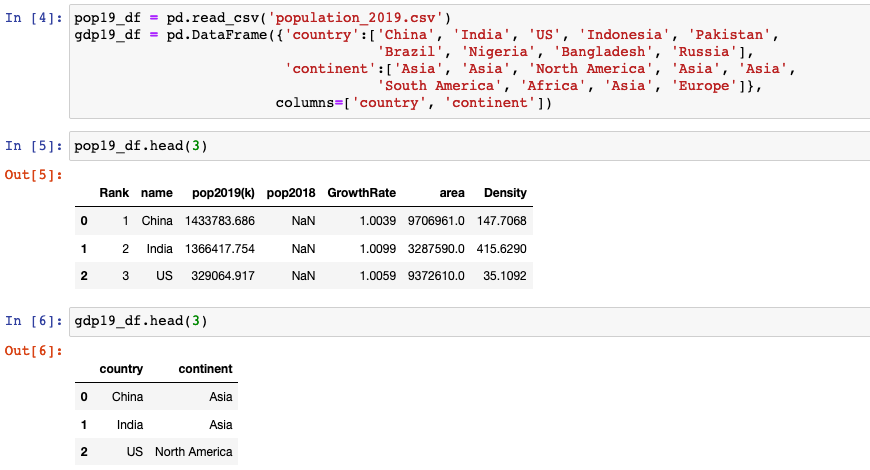## pyspark vs. pandas

### Checking dataframe size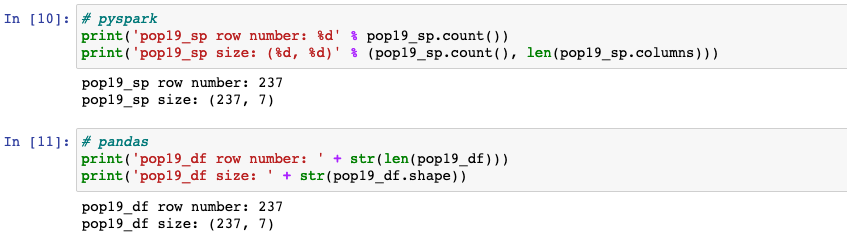• .count() counts the number of rows in pyspark.
• pandas.DataFrame.shape returns a tuple representing the dimensionality of the DataFrame.

### Checking unique values of a column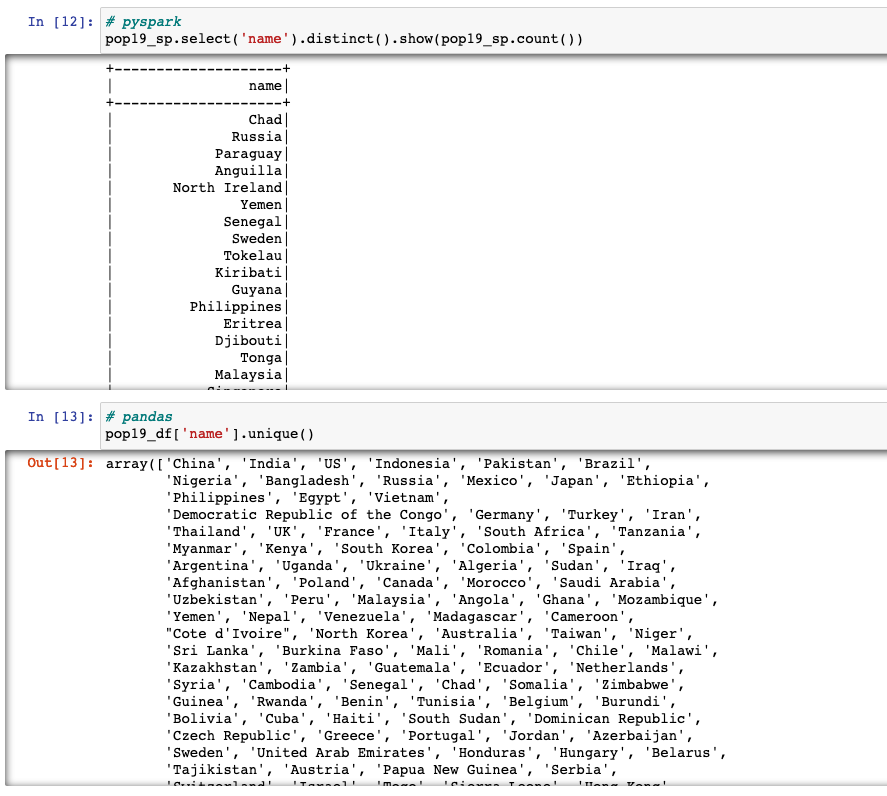• .select().distinct(): distinct value of the column in pyspark is obtained by using select() function along with distinct() function. select() takes up the column name as argument, followed by distinct() will give distinct value of the column.
• pandas.unique(): uniques are returned in order of appearance.

### Creating a new column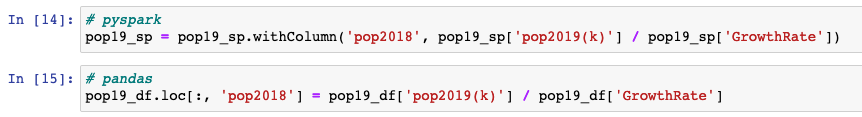• withColumn(colName, col) returns a new dataframe by adding a column or replacing the existing column that has the same name.

### Filtering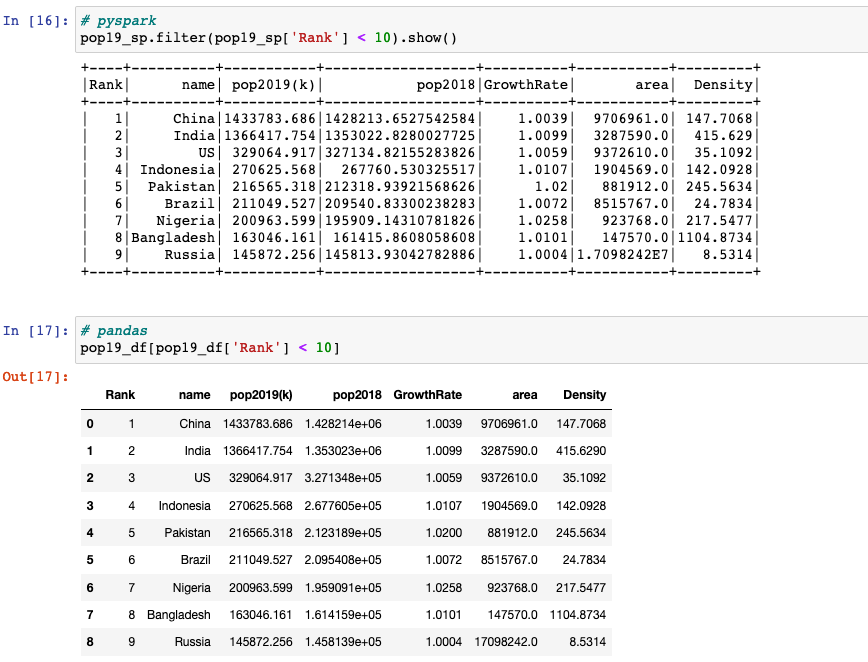• filter(condition) filters rows using the given condition.
• We can apply df[condition] to get only the rows that satisfy the condition.

### Selecting a list of columns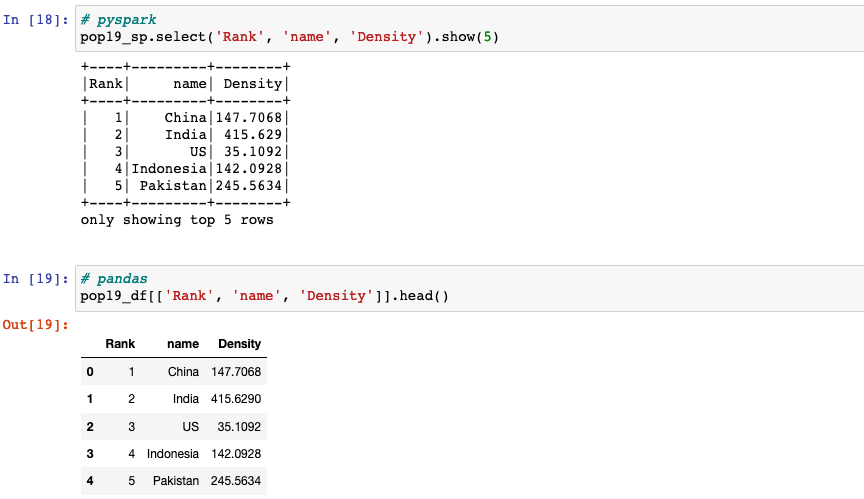• select(*cols) projects a set of expressions and returns a new DataFrame.
• [[*cols]]: we can pass a list of columns to [] to select columns in that order.

### Aggregating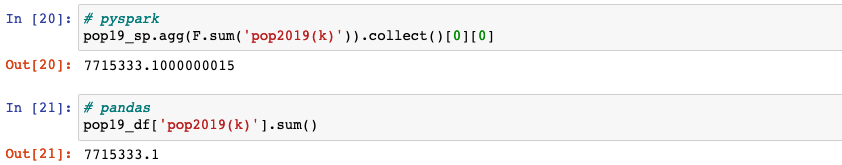• agg(*exprs): aggregates on the entire dataframe without groups (shorthand for df.groupBy.agg()).

### Renaming columns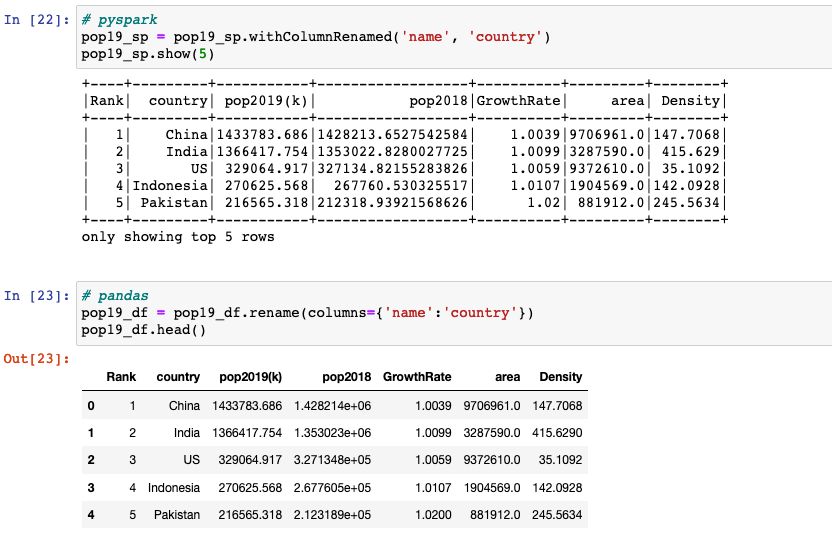• withColumnRenamed(existing, new) returns a new DataFrame by renaming an existing column. This is a no-op if the schema doesn’t contain the given column name.
• DataFrame.rename(**kwargs) alters axes labels.

### Joining 2 dataframes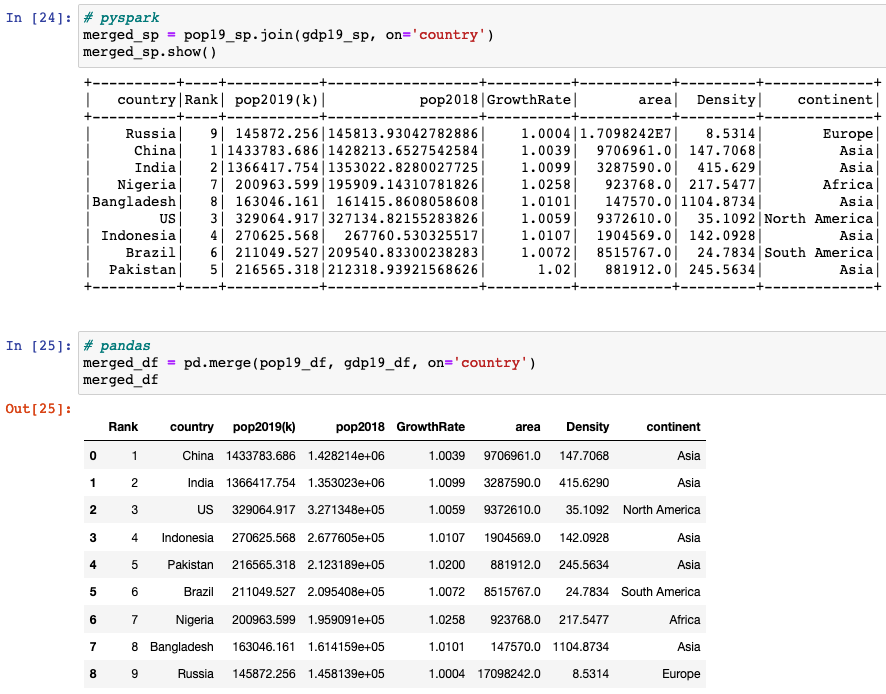• join(other, on=None, how=None) joins with another DataFrame, using the given join expression.
• DataFrame.merge(): merges DataFrame or named Series objects with a database-style join.

### Creating a new dataframe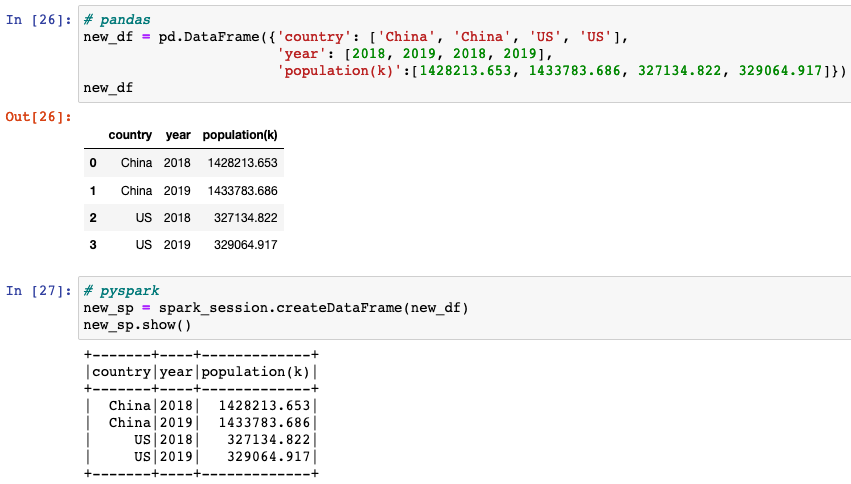• pandas.DataFrame() creates two-dimensional, size-mutable, potentially heterogeneous tabular data.
• createDataFrame() creates a dataframe from an RDD, a list or a pandas.DataFrame.

### Creating a pivot table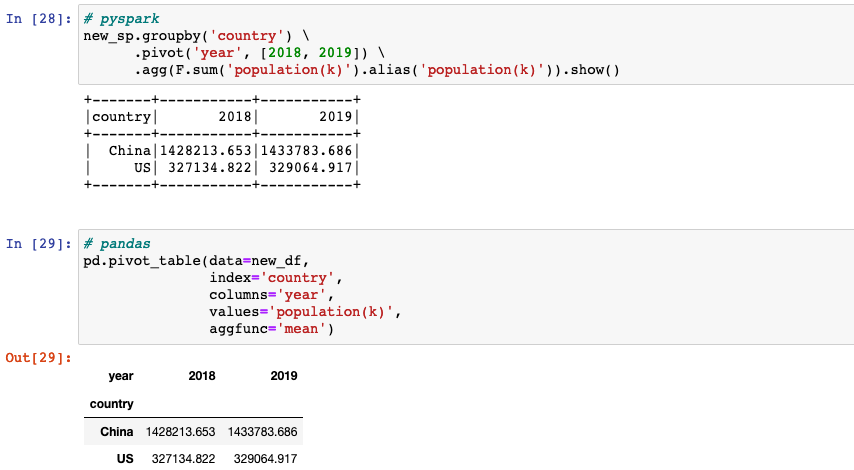• pivot(pivot_col, values=None): pivots a column of the current DataFrame and perform the specified aggregation.
• DataFrame.pivot_table() creates a spreadsheet-style pivot table as a dataframe.